# 分类：制作

## 只用两个舵机的的四足爬行机器人制作教程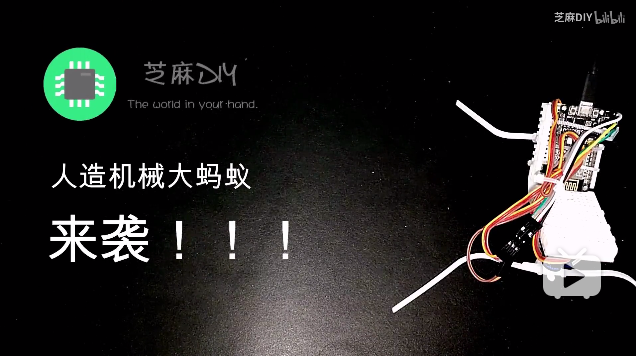## 来DIY一个空气质量指示器吧“衰荷滚玉闪晶光,一夜西风一夜凉。 雁阵声声蚊欲静,枣红点点桂流香。——《白露》（左河水）”白露过后，天气日渐转凉，转眼便已入秋了。

2012年，我国出台规定将用空气质量指数（AQI）替代原有的空气污染指数（API）。

## 使用STC15系列MCU控制舵机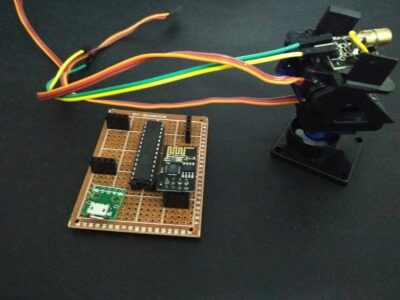## 制作:如何geek范儿的延长耳机使用时间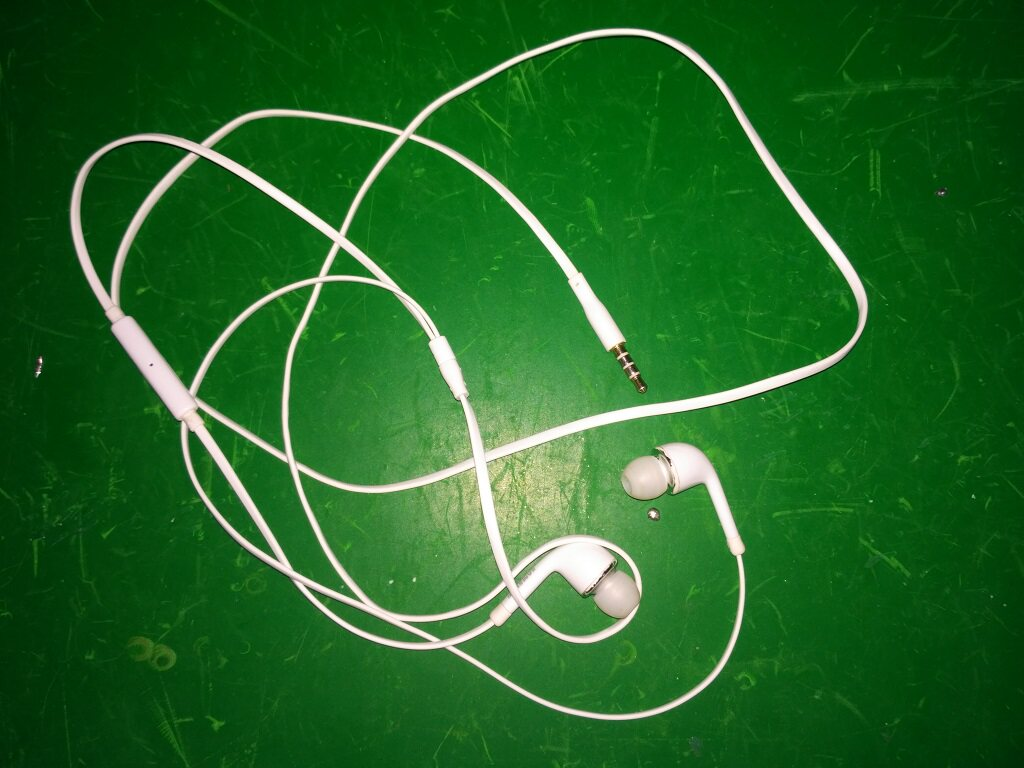## 安卓USB串口：单片机的新玩法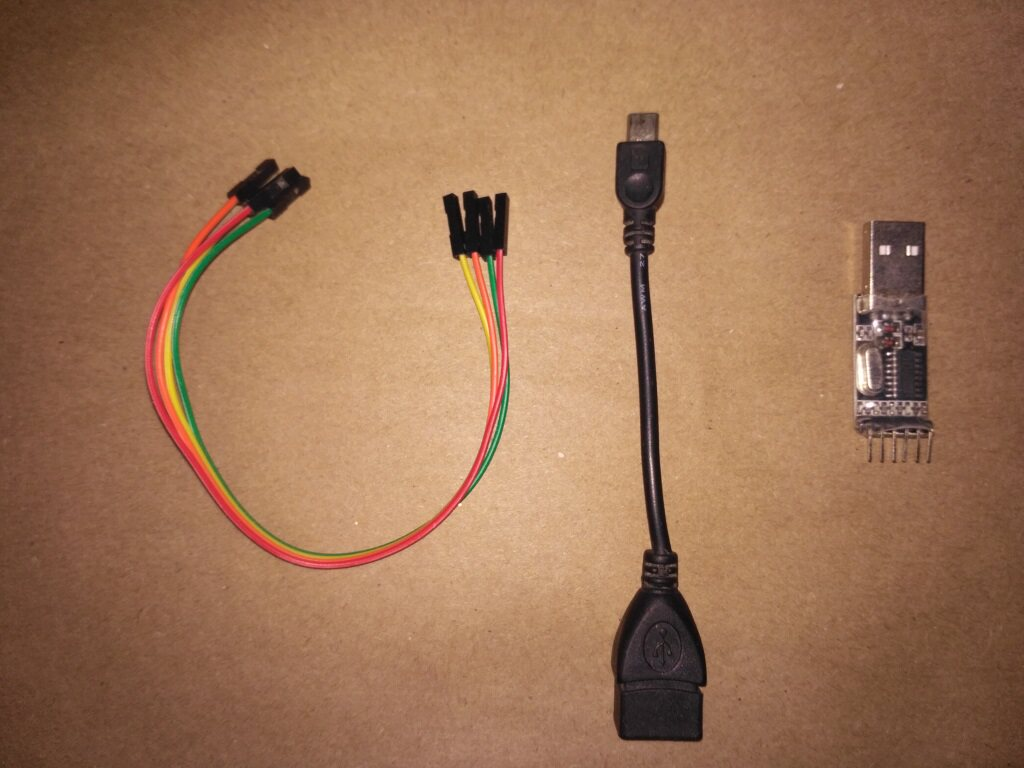## 制作：安卓手机遥控小车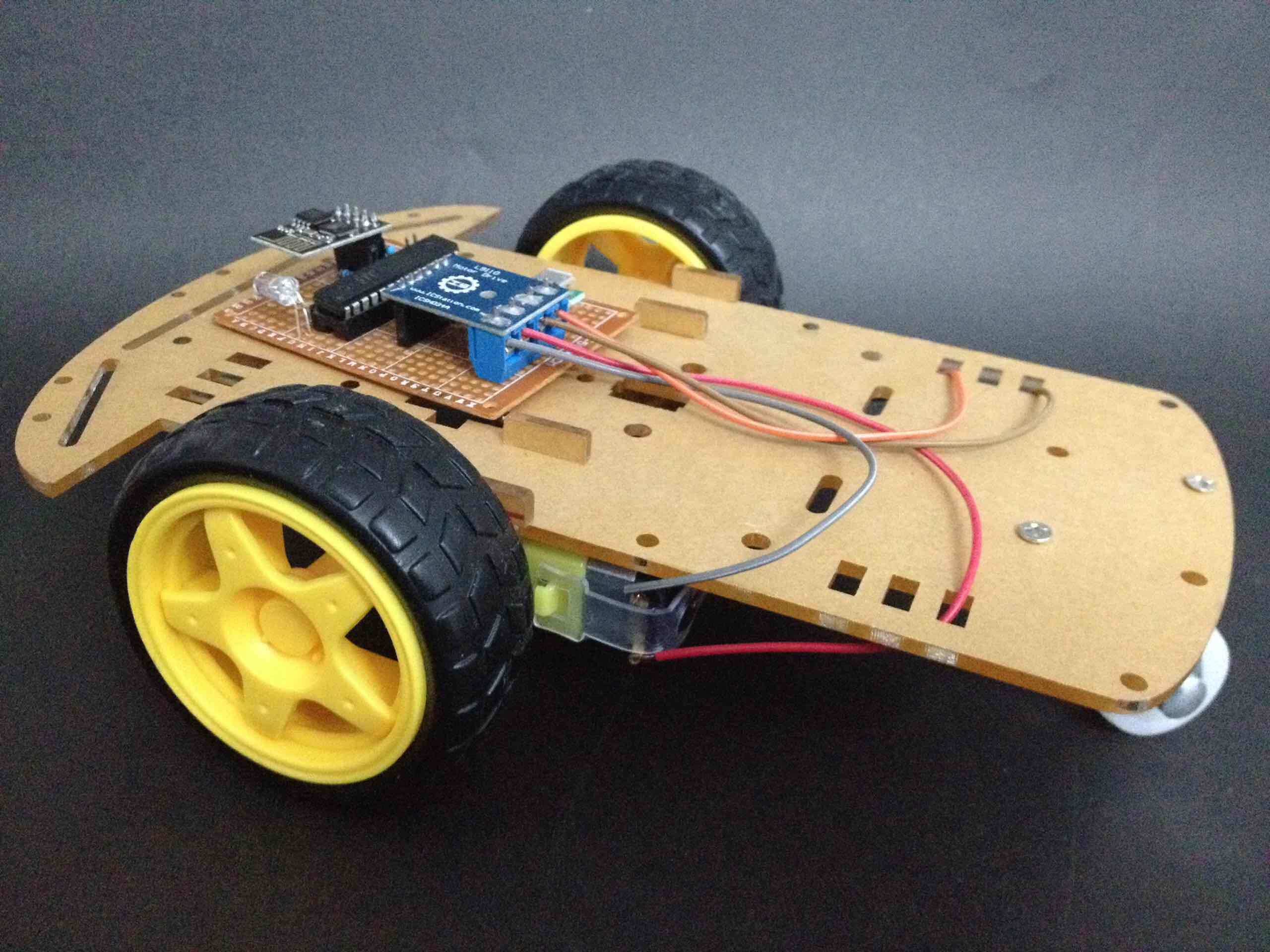## 简易的单片机时钟

``` #include #include //unsigned char table[]={0xc0,0xf9,0xa4,0xb0,0x99,0x92,0x82,0xf8,0x80,0x90}; //共阳数码管数字码 unsigned char table[]={0x3f,0x06,0x5b,0x4f,0x66,0x6d,0x7d,0x07,0x7f,0x6f}; //共阴数码管数字码 int time=2115,qian,bai,shi,ge,miao,num,time_shi=22,time_fen=31;```

``` void delay() {int a=500; while(a--); } void display() //数码管动态扫描 { qian=time_shi/10; //数码管个位 bai=time_shi%10; //十位 shi=time_fen/10; //百位 ge=time_fen%10; //千位 P0=table[ge]; P2=0xf7; delay(); P2=0xff; P0=table[shi]; P2=0xfb; delay(); P2=0xff; P0=table[bai]; P2=0xfd; delay(); P2=0xff; P0=table[qian]; P2=0xfe; delay(); P2=0xff; } void main() { TMOD = 0x21; SCON = 0x50; TH1 = 0xd0; TL1 = TH1; EA = 1; ET0 = 1; TI=1; ES = 1; TR1 = 1; TH0=(65536-45872)/256; //定时器初始化 TL0=(65536-45872)%256; TR0 = 1; while(1) { display(); if (time_fen==60) //如果分=60,时+1 {time_shi++; time_fen=0;} if(time_shi==24) //如果时=24,分时归零 { time_shi=0; time_fen=0; } } } void inter() interrupt 1 //定时器中断 { TH0=(65536-46083)/256; TL0=(65536-46083)%256; num++; if(num==20) { num=0; miao++; P1=miao; if (miao==60) { time_fen++; miao=0; } } } ```

```void UARTInterrupt(void) interrupt 4 { if(RI) { RI = 0; if(SBUF==1){time_shi++;} //收到‘1’，时+1 if(SBUF==2){time_fen++;} //收到‘2’，分+1 ES=0; TI=1; printf("NOW TIME IS %d : %d \n",time_shi,time_fen); //当串口收到信息时，输出消息 ES=1; TI=0; } else TI=0; }```

## 和你的单片机聊天## 嗒嗒：一个闪彩光的瓶子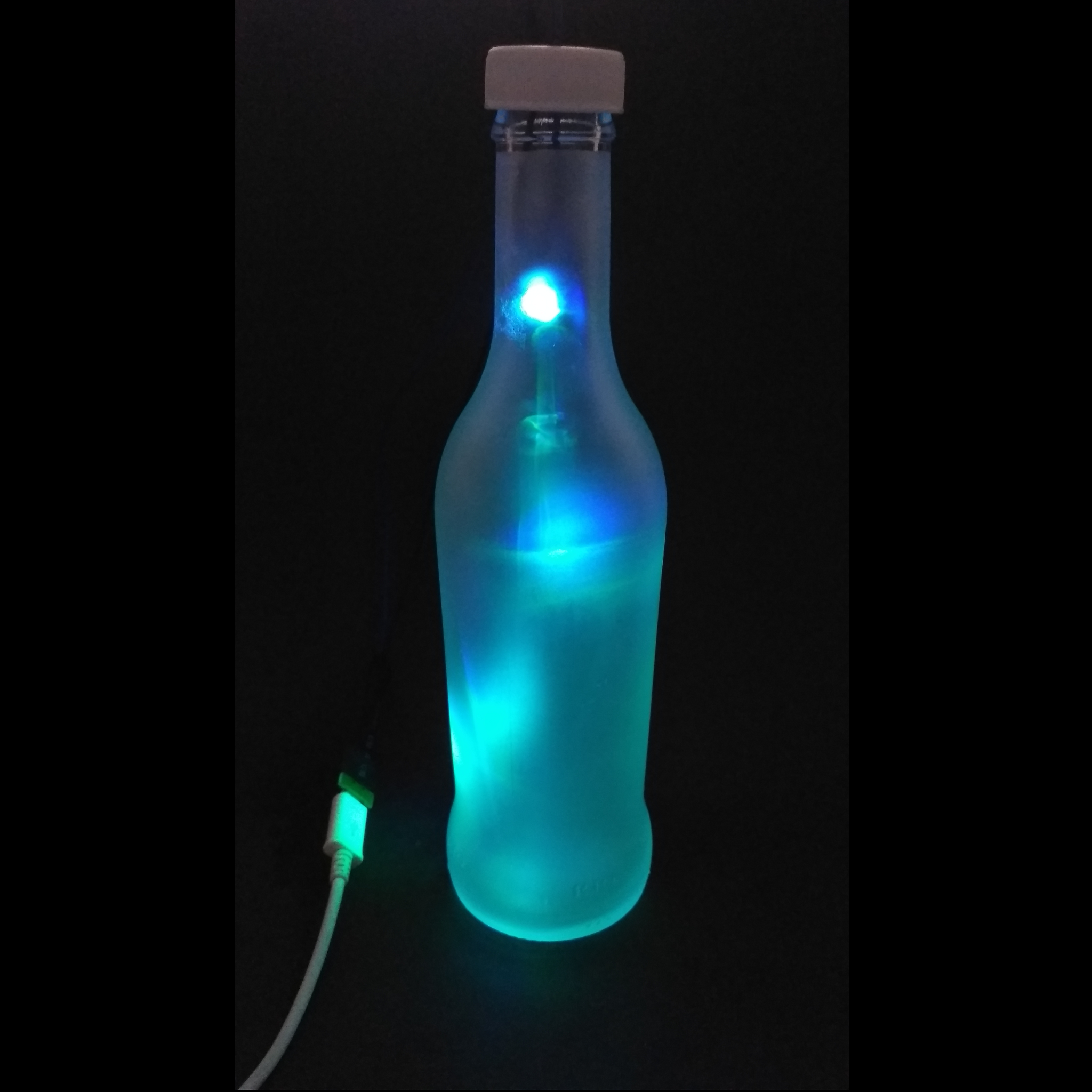是不是很好看呢？制作的过程是十分简单的。